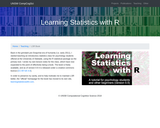Updating search results...

# 1 Result

View
Selected filters:
• University of New South WalesConditional Remix & Share Permitted
CC BY-SA
Rating
0.0 stars

Learning Statistics with R covers the contents of an introductory statistics class, as typically taught to undergraduate psychology students, focusing on the use of the R statistical software. The book discusses how to get started in R as well as giving an introduction to data manipulation and writing scripts. From a statistical perspective, the book discusses descriptive statistics and graphing first, followed by chapters on probability theory, sampling and estimation, and null hypothesis testing. After introducing the theory, the book covers the analysis of contingency tables, t-tests, ANOVAs and regression. Bayesian statistics are covered at the end of the book.

Subject:
Psychology
Social Science
Material Type:
Textbook
Provider:
University of New South Wales
Author:
Danielle Navarro